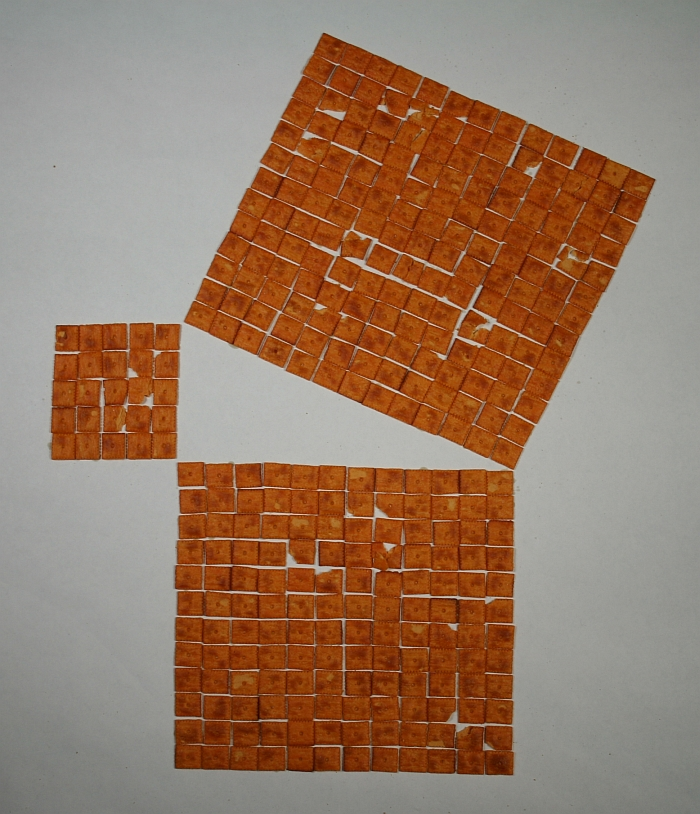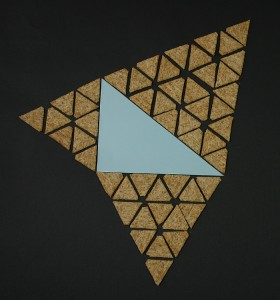### by George Hart for the Museum of Mathematics

In a right triangle with legs of length A and B, and hypotenuse of length C, the Pythagorean theorem tells us

A2 + B2 = C2

This is Proposition 47 in the first book of Euclid’s Elements. It is often illustrated by constructing three squares on the sides of a right triangle.The theorem is illustrated above in the special case of a 5-12-13 right triangle, which is one Pythagorean triple with integer values:

52 + 122 = 25 + 144 = 169 = 132

In the above example, this means the total number of crackers in the two smaller squares (on the legs) equals the total number of crackers in the larger square (on the hypotenuse). Imagining mathematically ideal crackers, all of equal area, this is an illustration of the Pythagorean theorem: the area of the large square equals the sum of the areas of the two small squares.Pythagoras was specifically discussing squares, but Euclid showed (in Proposition 31 of Book 6 of the Elements) that the theorem generalizes to any plane shape.  For example, if one constructs an equilateral triangle on the hypotenuse of a right triangle, its area is equal to the sum of the areas of two smaller equilateral triangles constructed on the legs.  That theorem is illustrated above with triangular crackers for the case of a 3-4-5 right triangle.

32 + 42 = 9 + 16 = 25 = 52

The first thing to notice is that the triangle of edge length three contains nine crackers, the triangle of edge length four contains sixteen crackers, and the triangle of edge length five contains twenty five crackers.  This squaring relationship between length and area is not specific to squares. We say nine is “three squared” but the picture shows that we could just as well say nine is “three triangled.”  Or, in fact, we could say “three pentagoned.”  It is true for any plane figure that if you scale its length by n then the area is scaled by n2. (I’m happy to stick with the traditional term “squared.”)So in general one can choose any plane shape and make three similar copies of it scaled to fit on the sides of a right triangle. It is easy to prove that the area of the large one is equal to the sum of the areas of the smaller ones.  In the example above, the blue triangle is a right triangle and the three squiggly shapes are similar, so the area of the red one is equal to the sum of the areas of the two green ones.  They don’t make crackers in this shape, but if I baked my own, I’d know that the areas work out. This is because the small cracker has area proportional to A2, let’s call its area kA2 (for some scaling factor, k). The medium-size cracker has length B/A times the length of the small one, so its area is scaled by (B/A)2, and so its area is kB2.  Similarly, the large one has area kC2. We easily prove that kA2 + kB2 = kC2 just by multiplying the Pythagorean Theorem through by k.

For an introduction to the Pythagorean Theorem and two proofs of its correctness, see Steven Strogatz’s “Square Dancing” column from March 14, 2010, in the NY Times. For more hands-on math demonstrations, see our past Math Monday columns for Make: Online.